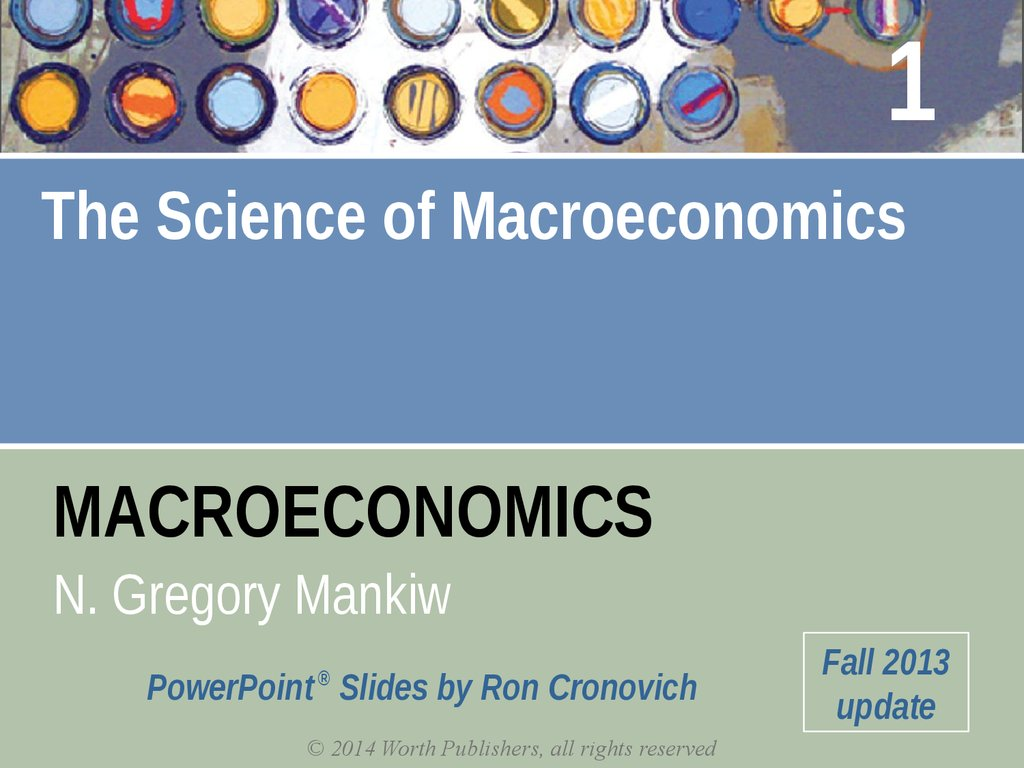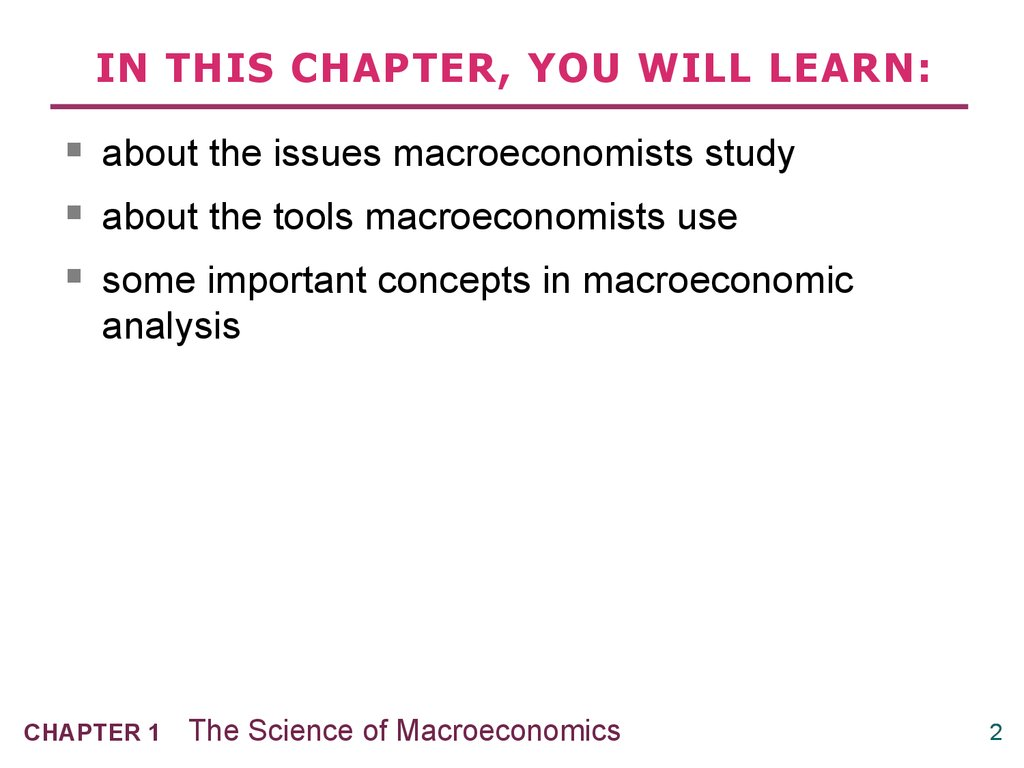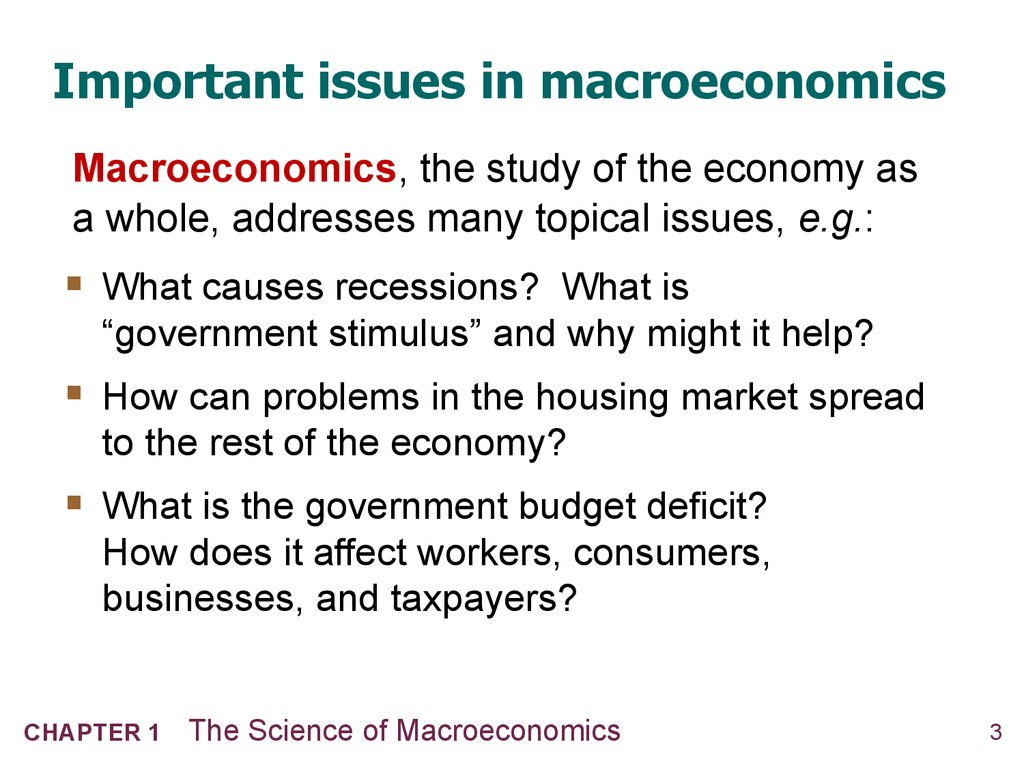# The Science of Macroeconomics

## 1.

1
The Science of Macroeconomics
MACROECONOMICS
N. Gregory Mankiw
®
PowerPoint Slides by Ron Cronovich
Fall 2013
update

## 2. IN THIS CHAPTER, YOU WILL LEARN:

about the issues macroeconomists study
about the tools macroeconomists use
some important concepts in macroeconomic
analysis
CHAPTER 1
The Science of Macroeconomics
2

## 3. Important issues in macroeconomics

Macroeconomics, the study of the economy as
a whole, addresses many topical issues, e.g.:
What causes recessions? What is
“government stimulus” and why might it help?
How can problems in the housing market spread
to the rest of the economy?
What is the government budget deficit?
How does it affect workers, consumers,
CHAPTER 1
The Science of Macroeconomics
3

## 4. Important issues in macroeconomics

Macroeconomics, the study of the economy as
a whole, addresses many topical issues, e.g.:
Why does the cost of living keep rising?
Why are so many countries poor? What policies
might help them grow out of poverty?
What is the trade deficit? How does it affect the
country’s well-being?
CHAPTER 1
The Science of Macroeconomics
4

## 5.

microeconomics Examines the functioning of individual industries and
the behavior of individual decision-making units—firms and households.
macroeconomics Deals with the economy as a whole.
Macroeconomics focuses on the determinants of total national income,
deals with aggregates such as aggregate consumption and investment,
and looks at the overall level of prices instead of individual prices.
aggregate behavior The behavior of all households and firms together.
CHAPTER 1
The Science of Macroeconomics
5

## 6.

Macroeconomic Concerns
Three of the major concerns of macroeconomics are
Output growth
Unemployment
Inflation and deflation
CHAPTER 1
The Science of Macroeconomics
6

## 7.

Macroeconomic Concerns
Output Growth
business cycle The cycle of short-term ups and downs in the
economy.
aggregate output The total quantity of goods and services produced
in an economy in a given period.
recession A period during which aggregate output declines.
Conventionally, a period in which aggregate output declines for two
consecutive quarters.
depression A prolonged and deep recession.
CHAPTER 1
The Science of Macroeconomics
7

## 8.

Macroeconomic Concerns
Output Growth
FIGURE 5.1 A Typical
In this business cycle, the
economy is expanding as it
moves through point A from
the trough to the peak.
When the economy moves
from a peak down to a trough,
through point B, the economy
is in recession.
CHAPTER 1
The Science of Macroeconomics
8

## 9. U.S. Real GDP per capita (2005 dollars)

\$50 000
9/11/2001
\$40 000
Great
Depression
\$30 000
\$20 000
First
oil price
shock
World
War I
Financial
crisis
Second oil
price shock
\$10 000
2010
2000
1990
1980
The Science of Macroeconomics
1970
1960
1950
1940
1930
CHAPTER 1
1920
1900
\$0
1910
World War II
9

## 10.

Macroeconomic Concerns
Unemployment
unemployment rate The percentage of the labor force that is
unemployed.
CHAPTER 1
The Science of Macroeconomics
10

## 11. U.S. Unemployment Rate (% of labor force)

30
Great
Depression
25
20
World
War I
15
World
War II
10
Oil price
shocks
Financial
crisis
2010
2000
1990
1980
The Science of Macroeconomics
1970
1960
1950
1940
1930
CHAPTER 1
1920
1900
0
1910
5
11

## 12.

Macroeconomic Concerns
Inflation and Deflation
inflation An increase in the overall price level.
hyperinflation A period of very rapid increases in the overall price
level.
deflation A decrease in the overall price level.
CHAPTER 1
The Science of Macroeconomics
12

## 13. U.S. Inflation Rate (% per year)

25
World
War I
20
Second
oil price
shock
First
oil price
shock
15
10
5
0
-5
2010
2000
1990
1980
The Science of Macroeconomics
1970
1960
1950
1940
1930
CHAPTER 1
1920
1900
1910
-10
-15
Financial
crisis
Great
Depression
13

## 14.

The Components of the Macroeconomy
Understanding how the macroeconomy works can be challenging because a
great deal is going on at one time. Everything seems to affect everything else.
To see the big picture, it is helpful to divide the participants in the economy into
(1) Households.
(2) Firms.
(3) The government.
(4) The rest of the world.
CHAPTER 1
The Science of Macroeconomics
14

## 15. Economic models

…are simplified versions of a more complex reality
irrelevant details are stripped away
…are used to
show relationships between variables
explain the economy’s behavior
devise policies to improve economic
performance
CHAPTER 1
The Science of Macroeconomics
15

## 16. Endogenous vs. exogenous variables

The values of endogenous variables
are determined in the model.
The values of exogenous variables
are determined outside the model:
the model takes their values and behavior
as given.
In the model of supply & demand for cars,
endogenous: P, Q d, Q s
exogenous: Y, Ps
CHAPTER 1
The Science of Macroeconomics
16

## 17. Example of a model: Supply & demand for new cars

Example of a model:
Supply & demand for new cars
shows how various events affect price and
quantity of cars
assumes the market is competitive: each buyer
and seller is too small to affect the market price
Variables
Qd = quantity of cars that buyers demand
Qs = quantity that producers supply
P = price of new cars
Y = aggregate income
Ps = price of steel (an input)
CHAPTER 1
The Science of Macroeconomics
17

## 18. The demand for cars

demand equation: Q d = D (P,Y )
shows that the quantity of cars consumers
demand is related to the price of cars and
aggregate income
CHAPTER 1
The Science of Macroeconomics
18

## 19. Digression: functional notation

General functional notation
shows only that the variables are related.
Q d = D (P,Y )
A specific functional form shows
A list of
the
the precise
quantitative
relationship.
variables
Example:
that affect Q d
D (P,Y ) = 60 – 10P + 2Y
CHAPTER 1
The Science of Macroeconomics
19

## 20. The market for cars: Demand

demand equation:
Q = D (P,Y )
d
P
Price
of cars
The demand curve
shows the relationship
between quantity
demanded and price,
other things equal.
CHAPTER 1
The Science of Macroeconomics
D
Q
Quantity
of cars
20

## 21. The market for cars: Supply

supply equation:
Q = S (P,PS )
s
P
Price
of cars
The supply curve
shows the relationship
between quantity
supplied and price,
other things equal.
CHAPTER 1
The Science of Macroeconomics
S
D
Q
Quantity
of cars
21

## 22. The market for cars: Equilibrium

P
Price
of cars
S
equilibrium
price
D
Q
equilibrium
quantity
CHAPTER 1
The Science of Macroeconomics
Quantity
of cars
22

## 23. The effects of an increase in income

demand equation:
Q d = D (P,Y )
An increase in income
increases the quantity
of cars consumers
demand at each price…
P
Price
of cars
P2
P1
…which increases
the equilibrium price
and quantity.
CHAPTER 1
S
The Science of Macroeconomics
D1
Q1 Q2
D2
Q
Quantity
of cars
23

## 24. The effects of a steel price increase

supply equation:
Q s = S ( P, P S )
P
Price
of cars
An increase in Ps
reduces the quantity of
cars producers supply
at each price…
S1
P2
P1
…which increases the
market price and
reduces the quantity.
CHAPTER 1
S2
The Science of Macroeconomics
D
Q2 Q1
Q
Quantity
of cars
24

## 25. NOW YOU TRY Supply and Demand

1. Write down demand and supply equations for
smartphones; include two exogenous variables
in each equation.
2. Draw a supply-demand graph for smartphones.
3. Use your graph to show how a change in one
of your exogenous variables affects the
model’s endogenous variables.
CHAPTER 1
The Science of Macroeconomics
25

## 26. The use of multiple models

No one model can address all the issues we
E.g., our supply-demand model of the car
market…
can tell us how a fall in aggregate income
affects price & quantity of cars.
cannot tell us why aggregate income falls.
CHAPTER 1
The Science of Macroeconomics
26

## 27. The use of multiple models

So we will learn different models for studying
different issues (e.g., unemployment, inflation,
long-run growth).
For each new model, you should keep track of
its assumptions
which variables are endogenous,
which are exogenous
the questions it can help us understand,
those it cannot
CHAPTER 1
The Science of Macroeconomics
27

## 28. Prices: flexible vs. sticky

Market clearing: An assumption that prices are
flexible, adjust to equate supply and demand.
In the short run, many prices are sticky –
adjust sluggishly in response to changes in
supply or demand. For example:
many labor contracts fix the nominal wage
for a year or longer
many magazine publishers change prices
only once every 3 to 4 years
CHAPTER 1
The Science of Macroeconomics
28

## 29. Prices: flexible vs. sticky

The economy’s behavior depends partly on
whether prices are sticky or flexible:
If prices sticky (short run),
demand may not equal supply, which explains:
unemployment (excess supply of labor)
why firms cannot always sell all the goods
they produce
If prices flexible (long run), markets clear and
economy behaves very differently
CHAPTER 1
The Science of Macroeconomics
29

## 30. Outline of this book:

Introductory material (Chaps. 1, 2)
Classical Theory (Chaps. 3–7)
How the economy works in the long run, when
prices are flexible
Growth Theory (Chaps. 8, 9)
The standard of living and its growth rate over the
very long run
Business Cycle Theory (Chaps. 10–14)
How the economy works in the short run, when
prices are sticky
CHAPTER 1
The Science of Macroeconomics
30

## 31. Outline of this book:

Macroeconomic theory (Chaps. 15–17)
Macroeconomic dynamics, models of consumer
behavior, theories of firms’ investment decisions
Macroeconomic policy (Chaps. 18–20)
Stabilization policy, government debt and
deficits, financial crises
CHAPTER 1
The Science of Macroeconomics
31

## 32. CHAPTER SUMMARY

Macroeconomics is the study of the economy as a
whole, including
growth in incomes
changes in the overall level of prices
the unemployment rate
Macroeconomists attempt to explain the economy
and to devise policies to improve its performance.
CHAPTER 1
The Science of Macroeconomics
32

## 33. CHAPTER SUMMARY

Economists use different models to examine
different issues.
Models with flexible prices describe the economy
in the long run; models with sticky prices describe
the economy in the short run.
Macroeconomic events and performance arise
from many microeconomic transactions, so
macroeconomics uses many of the tools of
microeconomics.
CHAPTER 1
The Science of Macroeconomics
33